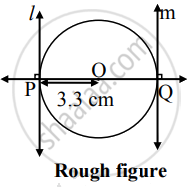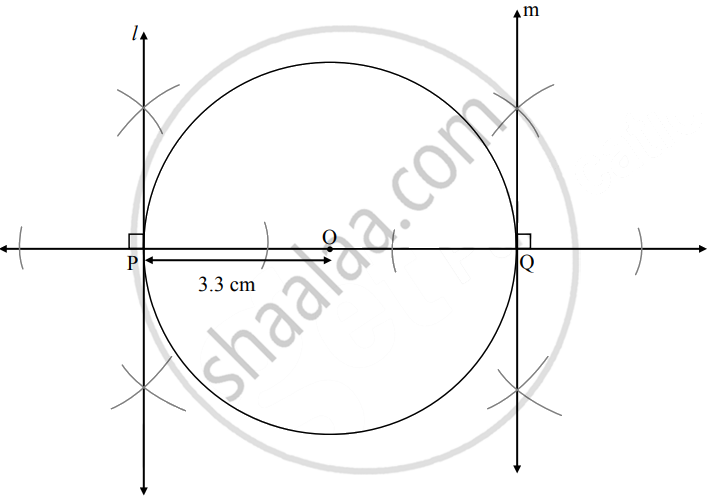# Draw a circle with radius 3.3 cm. Draw a chord PQ of length 6.6 cm. Draw tangents to the circle at points P and Q. Write your observation about the tangents - Geometry

Diagram

Draw a circle with radius 3.3 cm. Draw a chord PQ of length 6.6 cm. Draw tangents to the circle at points P and Q. Write your observation about the tangents

#### SolutionAnalysis:Steps of construction:

1. With center O, draw a circle of radius 3.3 cm
2. Draw a chord PQ of length 6.6 cm
3. Draw rays OP and OQ
4. Draw line l ⊥ ray OP at point P
5. Draw line m ⊥ ray OQ at point Q.
Lines l and m are the required tangents to the circle.
∴ Diameter = 2 × 3.3 = 6.6 cm
∴ Chord PQ is the diameter of the circle.
∴ The tangents through points P and Q (endpoints of diameter) are parallel to each other.
Concept: Construction of a Tangent to the Circle at a Point on the Circle
Is there an error in this question or solution?

Share